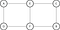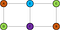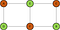# Dissecting Graph Coloring Problem

Graph Coloring is one of the interesting NP Complete problems. However, it is not just an interesting but challenging puzzle to solve; just like many classic computer science problems, it also has many real-life applications.

For instance, consider a scenario of a University exam. Students take many courses and it is important to ensure that no two courses with overlapping students have exams scheduled at the same time. On the contrary, if two courses do not have any common students, they can be scheduled at the same time. The question here is what is the minimum number of schedules required and which courses can have exams scheduled together! This scheduling problem is one of the real-world graph coloring problems. There are many more; if you are curious to know more about applications, you might like to watch this youtube video as well as this blogpost.

As this looks like the right time to formally introduce the graph coloring problem, let me do the honors.

Graph coloring problem is to color the graph vertices of a undirected graph with minimum number of colors such that no two adjacent (connected) vertices are colored same.

We can use greedy algorithm to color the graph satisfying the constraints that no two adjacent vertices have the same color. However, it doesn’t give the minimum number of colors.

Let’s get our hands dirty! Consider the graph below and try coloring it.A simple graph without coloring

If we start by coloring the vertices in the order A-B-C-D-E-F such that the restrictions are met, we get the graph below. This results in 4 colors.Graph colored “greedily” in the order A-B-C-D-E-F

Whereas, if we start from the top left corner in the order A-E-D-F-C-B such the restrictions are met, we get the graph below with just 2 colors (which is on of the optimum solutions)Graph colored “greedily” in the order A-E-D-F-C-B

Though we colored the graph in a greedy fashion, the order in which the coloring is done actually matters! Performing all the permutations on the order and performing the greedy algorithm will result in optimum solution(s). However, the order in which nodes can be colored is n!, where n is the number of vertices.

We can heuristically solve this using the lemma introduced in the paper Iterated Greedy Graph Coloring and the Difficulty Landscape.

After coloring the vertices once, group all the nodes together based on their colors. In the above example, after iteration 1 (if we have taken the initial order A-B-C-D-E-F), we get [A, B], [C, D], [E] & [F] based on the colors obtained after the first iteration. Now, permuting the nodes within the group will not produce any bad results. Why? This is because group i needs the maximum of what group i-1 takes, plus 1. This results in i colors for i groups. Similarly, permuting the groups (not individually) also will not cause any damage. This is because, coloring the nodes as 1,2,1,2,1,2 is same as 2,1,2,1,2,1. To clarify, taking permutations B-A-D-C-E-F is valid and will produce as good a result as A-B-C-D-E-F. Here [A, B] & [D, C] and permuted within the group, and groups [E] and [F] are permuted groups. Please note that [B, E, C, F, A, D] is not valid.

This approach reduces a lot of permutations. The paper suggests a couple of methods of permutation

• Vertices within groups to be permuted based on increasing and decreasing order of degrees
• Group permutation based on increasing and decreasing cardinality
• Random permutation of groups/vertices in individual groups
• A probabilistic combination of all the above methods in different iterations

This method uses the best (minimal) coloring identified till that time to order the nodes for coloring in the next iteration, which is guaranteed to produce results as good or better than the previous results. In optimization language, this tremendously prunes the search space!

This method, being run for long iterations has proved to produce good results in graph coloring problems.

Hope you had a gist of the graph coloring problem, its relevance, complexity, and one effective heuristic solution. Please let me know your inputs, suggestions, and questions. Feel free to reach out with any questions about the implementation. Happy coding!

Bincy Narath is a Data scientist professional. Her research interest spans from algorithm development to using machine learning and statistical techniques.

## More from Bincy Narath

Bincy Narath is a Data scientist professional. Her research interest spans from algorithm development to using machine learning and statistical techniques.